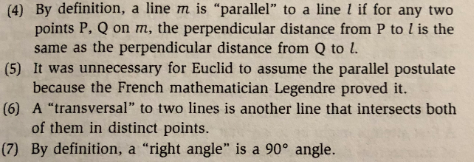(4) By definition, a line m is "parallel" to a line l if for any twopoints P, Q on m, the perpendicular distance from P to l is the(5) It was unnecessary for Euclid to assume the parallel postulate(6) A "transversal" to two lines is another line that intersects both(7) By definition, a "right angle" is a 90° angle.same as the perpendicular distance from Q to Lbecause the French mathematician Legendre proved it.of them in distinct points.

Question

State whether the following statements are correct or not:
Give a short explanation for each.help_outlineImage Transcriptionclose(4) By definition, a line m is "parallel" to a line l if for any two points P, Q on m, the perpendicular distance from P to l is the (5) It was unnecessary for Euclid to assume the parallel postulate (6) A "transversal" to two lines is another line that intersects both (7) By definition, a "right angle" is a 90° angle. same as the perpendicular distance from Q to L because the French mathematician Legendre proved it. of them in distinct points. fullscreen
Step 1

There are multiple questions in the image. So, I am answering the first question only, that is question number 4.

Please mention the exact question number in next query, so we can answer that question specifically.

Step 2

If the perpendicular distances of two points on a line from another line are equal(Assuming these two lines never intersect each other). Then, ...

Want to see the full answer?

See Solution

Want to see this answer and more?

Our solutions are written by experts, many with advanced degrees, and available 24/7

See Solution
Tagged in

Math